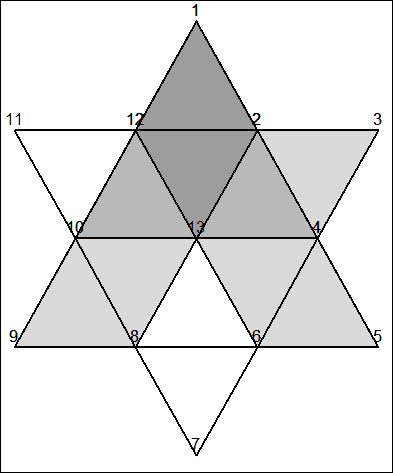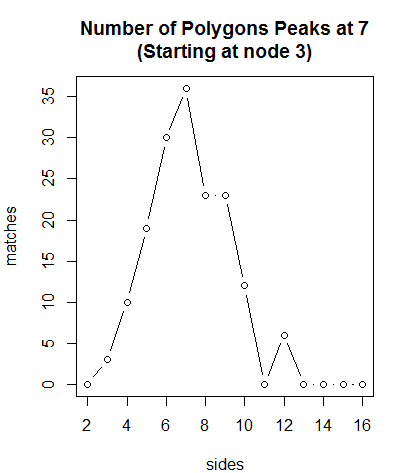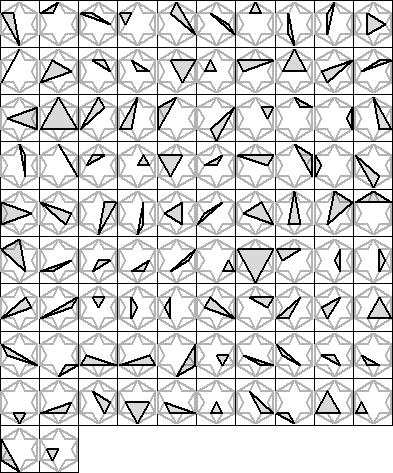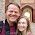## Tuesday, March 4, 2014

### The Star Puzzle

The Star Puzzle is a puzzle presented on The Math Forum.  I became aware of this problem by noticing the article and solution posted on Quantitative Decisions article section. It asks the question, "How many triangles, quadrilaterals, and irregular hexagons can we form from a star of David?"

In this post I will solve these questions using R as well as solve the more basic question, "How to we find the geometric placement of each of our nodes so as to graph all of our shapes perfectly?"

First off, we note that the star is constructed of two intersecting equilateral triangles which when connecting each intersection points creates a total of 12 smaller equilateral triangles.

Let us first define the origin as (0,0) and define the length of one of the larger sides as 9a (but for future reference just 9).  The a is there to indicate that we can arbitrarily scale the star at any time.

Now we want to find all of the nodes (corner pieces in all of the 12 triangles) on the star.  Some values we know already, for instance the x values are all -4.5, 0, or 4.5 because the star is centered at the origin and each side is 9.  Also, we know the length of each side of the smaller triangles since 3 smaller triangle lengths spans the larger triangle length thus length of each smaller triangle is 3, giving us the coordinates of our first two nodes (-3,0) and (3,0).

Next we need calculate the height of one of the triangles.  Using the Pythagorean Theorem.
3^2=1.5^2+b^2
b=(3^2-1.5^2)^.5=2.6

Now we can fill in all of the values.

Let us send these values to R.

```# First let us set the margins to be zero
par(mar = rep(0, 4))

# First we will come up with a node list
node <-
rbind(c(0,5.2),    c(1.5,2.6),  c(4.5,2.6),  c(3,0),
c(4.5,-2.6), c(1.5,-2.6), c(0,-5.2),   c(-1.5,-2.6),
c(-4.5,-2.6),c(-3,0),    c(-4.5,2.6),  c(-1.5,2.6),
c(0,0))

plot(node, type="n", ylim=c(min(node[,2]), max(node[,2])+.1))
for (i in 1:13) text(node[i,1],node[i,2]+.3, i)

# First the perimeter
lines(node[c(1:12,1),],lwd=2)

# Now the regular hexigon.
lines(node[c(seq(2,12,2),2),], lwd=2)

# Finally the lines connecting the intersection points.
lines(node[c(2,8),] , lwd=2)
lines(node[c(4,10),], lwd=2)
lines(node[c(6,12),], lwd=2)```

```

#####
# Now we are ready to try to solve the challenge. Let us first start by defining
# a list of connections for each node.

connections <- list(
c(12,2),         # for 1
c(1,12,13,4,3),  # for 2
c(2,4),          # for 3
c(2,3,5,6,13),   # for 4
c(4,6),          # for 5
c(7,8,13,4,5),   # for 6
c(8,6),          # for 7
c(9,10,13,6,7),  # for 8
c(8,10),         # for 9
c(11,12,13,8,9), # for 10
c(10,12),        # for 11
c(11,1,2,13,10), # for 12
c(2,4,6,8,10,12) # for 13
)

# In order to count the number of sides we will need to define a vector that
# collapses nodes on the same side.
collapser <- rbind(
c(1,4),
c(2,5),
c(3,6),
c(4,7),
c(5,8),
c(5,9),
c(6,9),
c(7,10),
c(8,11),
c(9,12),
c(10,1),
c(11,2),
c(12,3),
c(12,6),
c(2,8),
c(10,4))

# In the counting of sides.  If there is a single node between
# any of the above sets
# we will remove that node.  Thus 1,2,4 will become 1,4 which counts as one side.
# We only need to run the matching algorithm once because
# 1,2,4,5 -> drops 2 and 4 -> 1,5

# Let us create a function to count the number of sides.
nsides <- function(nodelist, collapser) {
# A list of nodes to keep
keeper <- rep(T,length(nodelist))
# Only evaluate sides if list of nodes >2
if (length(nodelist)>2) # Cycle through each set of 3 nodes
for (i in 1:(length(nodelist)))
for (ii in 1:nrow(collapser)) {
if (i+1 < length(nodelist)) {
if ((nodelist[i]==collapser[ii,1]&nodelist[i+2]==collapser[ii,2])|
(nodelist[i] ==collapser[ii,2]&nodelist[i+2]==collapser[ii,1]))
keeper[i+1] <- F
}
if (i+1 == length(nodelist))
if ((nodelist[i]==collapser[ii,1]&nodelist==collapser[ii,2])|
(nodelist[i] ==collapser[ii,2]&nodelist==collapser[ii,1]))
keeper[i+1] <- F
if (i == length(nodelist))
if ((nodelist[i]==collapser[ii,1]&nodelist==collapser[ii,2])|
(nodelist[i] ==collapser[ii,2]&nodelist==collapser[ii,1]))
keeper <- F
}
# Return both the list of condensed nodes and the number of sides
list(node=nodelist[keeper], sides=sum(keeper))
}

# Create a simple function for plotting node shapes
plot.node <- function(s, nodes=node, ntext=T, lwd=2, clear=F,
col = rgb(0, 0, 0,0.15),border="black", ...) {
if (clear) plot(nodes[s,], type="n", xaxt='n', yaxt='n',
ylim=c(min(nodes[s,2]), max(nodes[s,2])+.2), ...)
polygon(nodes[s,1],nodes[s,2],lwd=2, col = col, border=border)
if (ntext) for (i in s) text(nodes[i,1],nodes[i,2]+.3, i)
}

nsides(c(1,2,4,13,10,12),         collapser)
(s <- nsides(c(1,2,3,4,13,10,12), collapser))
plot.node(s[], clear=T, border="white")

(s <- nsides(c(12,1,2,4,5,6,13,12), collapser))
plot.node(s[])

(s <- nsides(c(1,2,13,8,9,10,12), collapser))
plot.node(s[])# Rotate matcher
rmatch <- function(first,second) {
for (i in 1:length(first)) {
mixer <- c(second[-(1:i)],second[1:i])
if (all(first==mixer)|all(rev(first)==mixer)) return(T)
}
F #
}

rmatch(1:5, c(2:5,1))
rmatch(5:1, c(2:5,1))
rmatch(5:1,1:5)

# We will use a recursive algorithm to search each node and each connection
# to each node.

nodesearch <- function(nodelist, connections, target.sides,
collapser, noisily=F) {
# Calculate number of side and shortest node list
fsides <- nsides(nodelist[-1], collapser)
# Calculate possible connections to the current node
cconct <- connections[[(rev(nodelist))]]
# Calculate available connections to current node given
# some nodes have already been used.
aconct <- cconct[!(cconct %in% nodelist[-1])]

if ((fsides[]>target.sides+1)|length(aconct)==0|
(nodelist==rev(nodelist)&length(nodelist)>1))
{

# If noisily option is selected then a print screen will occure any
# time there is a end of path.
if (noisily) {
print(nodelist)
print(paste("sides", fsides[]))
}

if (nodelist==rev(nodelist)&fsides[]==target.sides) {
# Search if the current solution is a duplicate of another
dup <- F
for (i in ret.ls) if (rmatch(fsides[], i)) dup <- T
if (!dup) ret.ls[[length(ret.ls)+1]] <<- rev(fsides[])
}
} else {
for (i in aconct) nodesearch(c(nodelist,i), connections, target.sides,
collapser,noisily)
}
}

# Stores the number of polygons starting at node 3 with a specific number
# of sides. We know there are no 2 sided polygons.
nodecount <- c(sides=2, matches=0)

# This will return all possible three sided objects
# (triangles in this case) which pass through node 3
sides <- 3; ret.ls <- list()
nodesearch(3, connections, sides, collapser)

# Create a counter of number of polygons including point 3
(nodecount <- rbind(nodecount, c(sides, length(ret.ls))))
plot.node(1:12, col=gray(1), ntext=F, clear=T)
for (i in ret.ls) plot.node(i, ntext=T, clear=F)
# 3 exist# Four sided
sides <- 4; ret.ls <- list()
nodesearch(3, connections, sides, collapser)
(nodecount <- rbind(nodecount, c(sides, length(ret.ls))))
plot.node(1:12, col=gray(1), ntext=F, clear=T)
for (i in ret.ls) plot.node(i, ntext=T, clear=F)
# 10 exist# Five sided
sides <- 5; ret.ls <- list()
nodesearch(3, connections, sides, collapser)
(nodecount <- rbind(nodecount, c(sides, length(ret.ls))))
plot.node(1:12, col=gray(1), ntext=F, clear=T)
for (i in ret.ls[1:2]) plot.node(i, ntext=T, clear=F)
# 19 exist

# Just plotting two of the irregular pentagons

# Six Sided
sides <- 6; ret.ls <- list()
nodesearch(3, connections, sides, collapser)
(nodecount <- rbind(nodecount, c(sides, length(ret.ls))))
plot.node(1:12, col=gray(1), ntext=F, clear=T)
for (i in ret.ls[1:2]) plot.node(i, ntext=T, clear=F)
# 30 exist

# Plotting two of the irregular hexigonsfor (sides in 7:16) {
ret.ls <- list()
nodesearch(3, connections, sides, collapser)
nodecount <- rbind(nodecount, c(sides, length(ret.ls)))
}
nodecount

par(mar = c(4,4,4,1))
plot(nodecount, type="b",
main="Number of Polygons Peaks at 7\n(Starting at node 3)")#########################
# Looking at all nodes
#########################

# Matrix for full node count
fnodecount <- c(sides=2, matches=0)

# We can collect all possible triangles:
sides <- 3; ret.ls <- list()
for (i in 1:13) nodesearch(i, connections, sides, collapser)
(fnodecount <- rbind(fnodecount, c(sides, length(ret.ls))))
# Which yeilds a list of 20 possible triangles

par(mfrow=c(4,5), mar=rep(0,4))
for (i in ret.ls) {
plot.node(1:12, col=gray(1), border=gray(.7), ntext=F, clear=T)
plot.node(i, ntext=F, clear=F)
}sides <- 4; ret.ls <- list()
for (i in 1:13) nodesearch(i, connections, sides, collapser)
(fnodecount <- rbind(fnodecount, c(sides, length(ret.ls))))

par(mfrow=c(6,5), mar=rep(0,4))
for (i in ret.ls) {
plot.node(1:12, col=gray(1), border=gray(.7), ntext=F, clear=T)
plot.node(i, ntext=F, clear=F)
}# pentagons
sides <- 5; ret.ls <- list()
for (i in 1:13) nodesearch(i, connections, sides, collapser)
(fnodecount <- rbind(fnodecount, c(sides, length(ret.ls))))
# 60 pentagons

# and hexigons
# pentagons
sides <- 6; ret.ls <- list()
for (i in 1:13) nodesearch(i, connections, sides, collapser)
(fnodecount <- rbind(fnodecount, c(sides, length(ret.ls))))
# 100 hexigons

par(mfrow=c(10,10), mar=rep(0,4))
for (i in ret.ls) {
plot.node(1:12, col=gray(1), border=gray(.7), ntext=F, clear=T)
plot.node(i, ntext=F, clear=F)
}# Notice that node one is used in the first three rows then node
# two is used in all other rows as well up till the last seven
# polygons.

# and septigons and more
for (sides in 7:16) {
ret.ls <- list()
for (i in 1:13) nodesearch(i, connections, sides, collapser)
print(fnodecount <- rbind(fnodecount, c(sides, length(ret.ls))))
}

par(mfrow=c(10,10), mar=rep(0,4))
plot(fnodecount, type="b",
main="Number of Polygons Peaks at 6")
lines(nnodecount, type="b", col="darkblue")# The blue lower line is the number of polygons that use node 3 as origin

# -----------------------------------

# Overall the code seems to work well.  The approach is easily generalizable and
# could calculate polygons even if nodes or connections were added or removed.

par(mar = c(0,0,0,0))
plot(node, type="n", ylim=c(min(node[,2]), max(node[,2])+.1))
for (i in 1:13) text(node[i,1],node[i,2]+.3, i)
lines(node[c(1:12,1),],lwd=2)
lines(node[c(seq(2,12,2),2),], lwd=2)
lines(node[c(2,8),] , lwd=2)
lines(node[c(4,10),], lwd=2)
lines(node[c(6,12),], lwd=2)

# Adding in the lines to the outer hexigon
lines(node[c(seq(1,11,2),1),], lwd=2)connections <- list(
c(12,2,11,3),    # for 1
c(1,12,13,4,3),  # for 2
c(2,4,1,5),      # for 3
c(2,3,5,6,13),   # for 4
c(4,6,3,7),      # for 5
c(7,8,13,4,5),   # for 6
c(8,6,5,9),      # for 7
c(9,10,13,6,7),  # for 8
c(8,10,11,7),    # for 9
c(11,12,13,8,9), # for 10
c(10,12,9,1),    # for 11
c(11,1,2,13,10), # for 12
c(2,4,6,8,10,12) # for 13
)

# Matrix for full node count
fnodecount <- c(sides=2, matches=0)

# Looking at possible triangles given our new connections:
sides <- 3; ret.ls <- list()
for (i in 1:13) nodesearch(i, connections, sides, collapser)
(fnodecount <- rbind(fnodecount, c(sides, length(ret.ls))))
# We get 92 possible triangles

par(mfrow=c(10,10), mar=rep(0,4))
for (i in ret.ls) {
plot.node(seq(1,11,2), col=gray(1), border=gray(.7), ntext=F, clear=T)
plot.node(1:12, col=gray(1), border=gray(.7), ntext=F, clear=F)
plot.node(i, ntext=F, clear=F)
}# I notice that plot row 2 col 1 and row 4 col 2 seems
# to be lines which means that there is some kind of error
# in the code somewhere.

# It is important to note that the more nodes/connections the
# more computationally complex the problem becomes.  If there
# were no node elimination and each node had the same number of
# connections then the computations (c) would be approximately
# equal to:

# Computations = k*c^s

# Where s is the number of sides and k is the number of computations
# within each search algorithm.

# With the star since the average number of connections is
# 3.7 for a triangle an
# approximation of the number of computations is

# k*3.7^4=187k

# However, adding the six connections increases the average number
# of connections by 6/13.

# k*(3.7+6/13)^4=300k

# Thus even adding relatively few connections significantly increases
# the processing time.

# k*3.7^5=693k

# With the new

# k*(3.7+6/13)^5=1248k

# This number is only an approximation because each iteration does
# decrease the number of possible connections.  However, each iteration
# also results in a longer list of matches that need to be check through
# before adding to the list of solutions, k()'>0.

sides <- 4; ret.ls <- list()
for (i in 1:13) nodesearch(i, connections, sides, collapser)
(fnodecount <- rbind(fnodecount, c(sides, length(ret.ls))))

# Yeilds 351 possible matches

Created by Pretty R at inside-R.org

```

1.1.